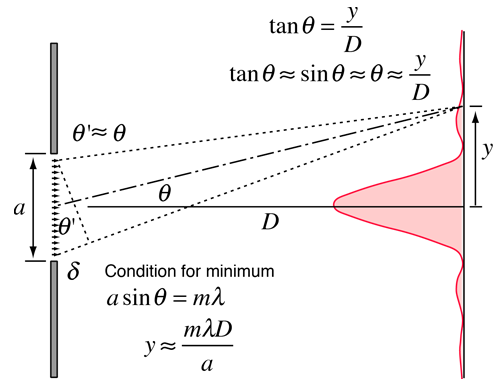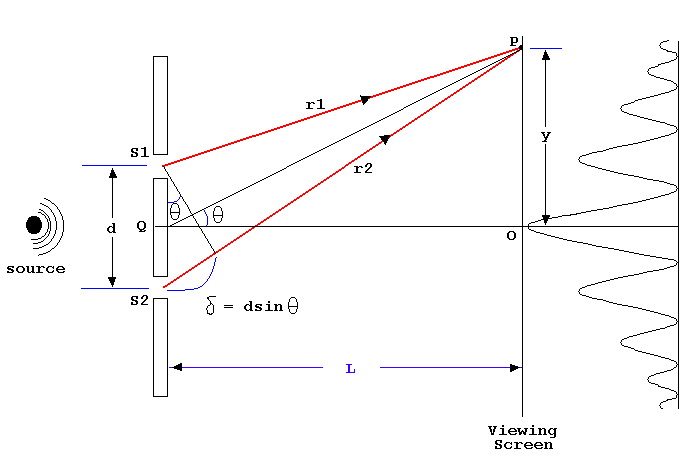Ravela Da Cruz

HS-PS4-3

double-slit experiment

single-slit diffraction formula

single-slit diffraction experiment

diffraction of light

# Double Slit Diffraction Study Guide

Double-slit diffraction, meaning showcases the effect of light passing through two slits that interact with each other.

Table of content

# INTRODUCTION

In May of 1801, while considering some of Newton’s experiments, Young came up with the fundamental idea for the double-slit experiment to exhibit the interference of light waves. The demonstration would deliver evidence that light was a wave, not a particle. Let us learn more about the double-slit experiment and understand double-slit diffraction.

## ABOUT THE CONDUCTION OF  THE DOUBLE SLIT EXPERIMENTSource

In the first version of the double slits experiment, Young didn’t use 2 slits but rather a single slim card. He covered a window with a piece of paper with a tiny hole in it. A thin beam of light passed through the hole. He held the card in the light beam, breaking the beam in two. Light passing on one side of the card interrupted light from the other side of the card, creating fringes, which Young observed on the opposite wall.

Young also used his data to calculate the wavelengths of different colors of light, coming very close to modern values.

## WHAT IS DOUBLE-SLIT DIFFRACTION?

• Double slit diffraction definition refers to an experiment in which light is permitted to diffract through slits that produce fringes or wave-like interference patterns on an opposite screen.
• Double-slit diffraction is the observed outcome where light passing through two slits produces beams that interact with each other.
• Analyzing the interference pattern and equations explains the observed phenomena with light passing through double-slits.

## CALCULATING THE WAVELENGTH FROM A DOUBLE-SLIT EXPERIMENTSource

To calculate the wavelength of the double-slit interference pattern, you need to measure a few distances. From the figure,

• L is the distance between 2 slits,
• The line in the middle is known as the central line;
• x is the length from the central line,
• d is the distance between two slits.
• The rest of the lines present are known as the fringes.

While experimenting, you can observe there are two triangles, a black triangle and a red triangle. If they are of the same ratio of corresponding sides, i.e., if x and L of the red triangle are the same as the x and L of the black triangle, then the double-slit diffraction formula is:

λ= xd / L

# SUMMARY

• Light is a wave and produces an interference pattern in the double-slit experiment.
• The interference pattern consists of alternative bright and dark lines; the bright lines are called fringes.
• In a double-slit experiment, the wavelength can be calculated using this equation: λ= xd / L.

## FAQs

Q. What does the double-slit experiment show?

The double-slit experiment is a demonstration that light and matter can display attributes of both classically defined waves and particles. It also shows the double-slit diffraction intensity.

Q. Is diffraction grating double slits?

A diffraction grating is a large number of evenly spaced and distributed parallel slits. The light passing through is diffracted in a pattern the same as a double slit, with bright regions at various angles.

Q. What happens when the slit width is doubled?

When the slit width is doubled, the energy falls per sec on the screen is doubled, but the angular width of the central maxima (Δθ=b2λ) is halved.

Q. What is the difference between single and double slits?

In single slit diffraction, light outstretches in a line perpendicular to the slit. But in double-slit diffraction, light diffracts when passing through the slits, but the light from those then interferes and produces an interference pattern on the screen. This is the double-slit diffraction pattern difference between single and double slits.

We hope you enjoyed studying this lesson and learned something cool about Double Slit Diffraction! Join our Discord community to get any questions you may have answered and to engage with other students just like you! We promise, it makes studying much more fun!�_

## SOURCES

1. Double-slit Diffraction: https://flexbooks.ck12.org/cbook/ck-12-physics-flexbook-2.0/section/13.8/primary/lesson/double-slit-interference-phys/ Accessed 14th April 2022.